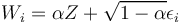# Monte Carlo Simulation of Credit Portfolios

## Definition

Monte Carlo simulation of Credit Portfolios is a computational method typically used for the calculation of Credit Value at Risk and economic capital for credit portfolios held by banks and similar institutions. The approach is an example of using Simulation Models for the purpose of establishing a Risk Distribution. Once the distribution is obtained it is possible to estimate various risk measures for the credit portfolio.

## Model Categories

There are several broad categories of models (which generally overlap) and very many variations in data inputs, model specification and model calibration:

## Standard Methodology

The following describes a standard one-period Credit VaR methodology for illustration purposes.

### Asset value simulation

The asset value simulation stage uses a factor model of asset values, with random specific error terms for individual assets that represent specific performance risk.

The factors represent the state of the economy or its sectors and can have different structures (hierarchical, quantitative driven).

The Monte Carlo simulation generates random sets of future values of the economic factors and of the specific risks, from which the asset values derive directly. The calculation is typically normalized: The main object of interest is not the expected asset return or its volatility, but the correlations between assets.

Generate standardized random normal distribution of the common factors Z (the number of factors may extend from one to many hundreds)

• Generate standardized random distributions of the specific residuals of all obligors (depends on the size of the portfolio)
• Calculate the resulting standardized random variable which is the asset value W of each obligor

Mathematically the obligor return is the weighted sum of specific and macro risk realizations (e.g.):$W_{i} = \alpha Z + \sqrt{1 - \alpha} \epsilon_{i}$

Once simulated asset returns are computed, the methodology proceeds to compute the credit status of exposures in the portfolio on a per scenario basis.

### Credit loss calculation

Credit losses due to migration or default are calculated per scenario basis for all exposures. In standardized Structural Credit Models asset values below certain thresholds trigger default. The procedure is as follows:

• Transform simulated obligor asset value, for each obligor, into a default 0-1 variable by comparing with a pre-calculated default threshold
• For valuation purposes, compute also migration states by comparing asset return with pre-calculated rating thresholds
• Calculate the losses for each obligor, given the default / migration variable and the aggregation of all exposures to that obligor
• Sum all losses to get one value for total portfolio loss in each simulation
• Repeat the simulation as many times as desired.

Aggregation of the results of a large number of trials generates a portfolio value Probability Distribution